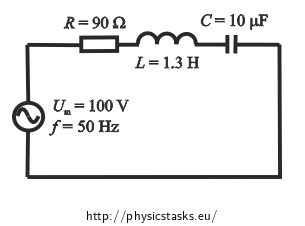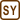## Instantaneous Voltage and Current Values in a Series RLC Circuit

An AC series circuit consists of a resistor with resistance of 90 Ω, a coil with inductance of 1.3 H and a capacitor with capacitance of 10 μF. The circuit is connected to an AC voltage source with amplitude of 100 V and frequency of 50 Hz. Write an equation for instantaneous values of voltage and current in the circuit, if the initial phase of the current is zero.• #### Given values

We write down the quantities and values given in the section Assignment

 Amplitude of the alternating voltage in the circuit Um = 100 V Frequency of the AC power source f  = 50 Hz Resistance of the resistor R = 90 Ω Inductance of the coil L = 1.3 H Capacitance of the capacitor C = 10 μF Initial current phase φ0i = 0

Quantities we want to determine:

 Instantaneous current value i(t) = ? (A) Instantaneous voltage value u(t) = ? (V)

• #### Hint 1

The behaviour of alternating voltage and current can be described by a sine function.

Find the equations describing the instantaneous values of the alternating voltage and the current.

• #### Hint 2

When evaluating these equations we need to know the current amplitude Im and a phase difference φ0u between the voltage and the current. These we determine by using Ohm's law for alternating current.

In the task Series RLC Circuit you can find a detailed explanation of the calculation of the current and the phase difference.

• #### Determining amplitude of current Im

We apply Ohm's law for a circuit with alternating current:

$I_m=\frac{U_m}{Z}=\frac{U_m}{\sqrt{R^2+\left(X_L-X_C\right)^2}}=\frac{U_m}{\sqrt{R^2+\left(2 \pi f L - \frac{1}{2 \pi f C}\right)^2}}$

We insert the given values:

$I_m=\frac{100}{\sqrt{90^2+\left(2 \pi \cdot 50 {\cdot} 1{.}3 - \frac{1}{2 \pi \cdot 50 {\cdot} 10 \cdot 10^{-6}}\right)^2}} \, \mathrm{A}$ $I_m \,\dot{=}\, 0{.}79 \, \mathrm{A}$
• #### Determining phase difference between voltage and current φ0u

We use the formula for phase difference expressed by impedance of each element.

$\mathrm{tg\,} \varphi_{0u} = \frac{X_L - X_C}{R}= \frac{\omega L- \frac{1}{ \omega C}}{R}= \frac{2 \pi f L- \frac{1}{ 2 \pi f C}}{R}$ $\varphi_{0u} = \mathrm{arctg\,}\left(\frac{2 \pi f L- \frac{1}{ 2 \pi f C}}{R}\right)$

Numerical solution:

$\varphi_{0u} = \mathrm{arctg\,}\left(\frac{2 \pi f L- \frac{1}{ 2 \pi f C}}{R}\right)= \mathrm{arctg\,}\left(\frac{2\pi \cdot 50 {\cdot} 1.3 - \frac{1}{ 2 \pi \cdot 50 {\cdot} 10 \cdot 10^{-6}}}{90}\right)$ $\varphi_{0u} \,\dot{=}\, 45 ^{\circ} = \frac{\pi}{4}$
• #### Instantaneous values of alternating voltage and current

Instantaneous value of alternating current:

The phase difference of the current is zero; the current amplitude Im has been calculated above.

$i(t)=I_m \, \sin(\omega t + \varphi_{0i})=I_m\, \sin( 2 \pi f t + \varphi_{0i} )$ $i(t)\,\dot{=}\,0.79 \cdot \sin(2 \pi 50 t)\,\mathrm A =0.79 \cdot \sin(100 \pi t)\,\mathrm A$

Instantaneous value of alternating voltage:

We know the voltage amplitude Um and we have determined the phase difference.

$u(t)=U_m \, \sin(\omega t + \varphi_{0u})=U_m \, \sin( 2 \pi f t + \varphi_{0u} )$ $u(t)\,\dot{=}\,100 \cdot \sin( 2 \pi 50 t + \frac{\pi}{4} )\,\mathrm V = 100\cdot \sin( 100 \pi t + \frac{\pi}{4} )\,\mathrm V$

The time values inserted into the equations are converted into seconds.

$i(t)=0{.}79 \cdot \sin\left({100 \pi t}\right)\,\mathrm A$ $u(t)=100 \cdot \sin\left( 100 \pi t + \frac{\pi}{4} \right)\,\mathrm V$